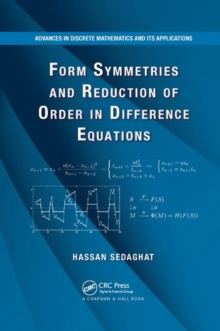Supporting your high street Find out how »
• My AccountForm Symmetries and Reduction of Order in Difference Equations Paperback / softback

Description

Form Symmetries and Reduction of Order in Difference Equations presents a new approach to the formulation and analysis of difference equations in which the underlying space is typically an algebraic group.

In some problems and applications, an additional algebraic or topological structure is assumed in order to define equations and obtain significant results about them.

Reflecting the author's past research experience, the majority of examples involve equations in finite dimensional Euclidean spaces. The book first introduces difference equations on groups, building a foundation for later chapters and illustrating the wide variety of possible formulations and interpretations of difference equations that occur in concrete contexts.

The author then proposes a systematic method of decomposition for recursive difference equations that uses a semiconjugate relation between maps.

Focusing on large classes of difference equations, he shows how to find the semiconjugate relations and accompanying factorizations of two difference equations with strictly lower orders.

The final chapter goes beyond semiconjugacy by extending the fundamental ideas based on form symmetries to nonrecursive difference equations. With numerous examples and exercises, this book is an ideal introduction to an exciting new domain in the area of difference equations.

It takes a fresh and all-inclusive look at difference equations and develops a systematic procedure for examining how these equations are constructed and solved.

Information

• Format: Paperback / softback
• Pages: 325 pages, 500+; 31 Illustrations, black and white
• Publisher: Taylor & Francis Ltd
• Publication Date:
• Category: Calculus & mathematical analysis
• ISBN: 9781138374126

£51.00

£45.59

on all orders

Pick up orders

from local bookshops